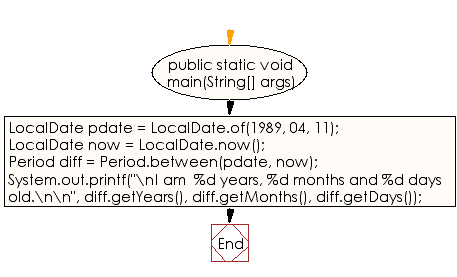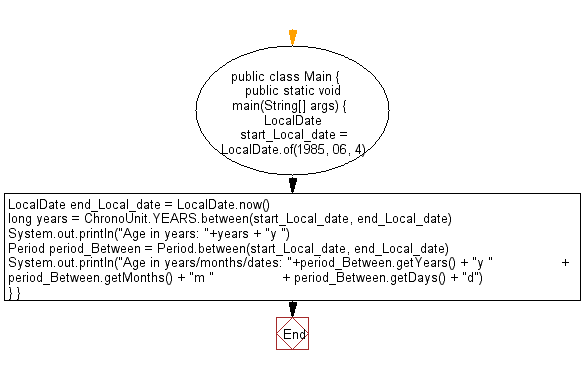﻿ Java exercises: Calculate your age - w3resource# Java DateTime, Calendar Exercises: Calculate your age

## Java DateTime, Calendar: Exercise-32 with Solution

Write a Java program to calculate your age.

Sample Solution:

Java Code:

``````import java.time.*;
import java.util.*;

public class Exercise32 {
public static void main(String[] args)
{
// date of birth
LocalDate pdate = LocalDate.of(1989, 04, 11);
// current date
LocalDate now = LocalDate.now();
// difference between current date and date of birth
Period diff = Period.between(pdate, now);

System.out.printf("\nI am  %d years, %d months and %d days old.\n\n",
diff.getYears(), diff.getMonths(), diff.getDays());
}
}
```
```

Sample Output:

```I am  28 years, 2 months and 10 days old.
```

N.B.: The result may varry for your system date and time.

Flowchart:Alternate Soluation:

Java Code:

``````//MIT License: https://bit.ly/35gZLa3
import java.time.LocalDate;
import java.time.Period;
import java.time.temporal.ChronoUnit;
import java.util.Calendar;
import java.util.Date;

public class Main {

public static void main(String[] args) {

LocalDate start_Local_date = LocalDate.of(1985, 06, 4);
LocalDate end_Local_date = LocalDate.now();

long years = ChronoUnit.YEARS.between(start_Local_date, end_Local_date);
System.out.println("Age in years: "+years + "y ");

Period period_Between = Period.between(start_Local_date, end_Local_date);
System.out.println("Age in years/months/dates: "+period_Between.getYears() + "y "
+ period_Between.getMonths() + "m "
+ period_Between.getDays() + "d");
}
}

``````

Sample Output:

```Age in years: 34y
Age in years/months/dates: 34y 5m 12d
```

Flowchart:Java Code Editor:

Improve this sample solution and post your code through Disqus

What is the difficulty level of this exercise?

﻿

## Java: Tips of the Day

Directory Content:

Java allows you to get the names of all subdirectories and files in a folder as an array, which can then be sequentially viewed.

```import java.io.*;

public class ListContents {
public static void main(String[] args) {
File file = new File("//home//user//Documents/");
String[] files = file.list();

System.out.println("Listing contents of " + file.getPath());
for(int i=0 ; i < files.length ; i++)
{
System.out.println(files[i]);
}
}
}
```